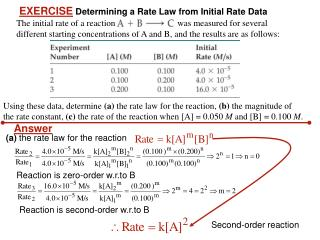DownloadDownload PresentationEXERCISE Determining a Rate Law from Initial Rate Data

# EXERCISE Determining a Rate Law from Initial Rate Data

Download Presentation## EXERCISE Determining a Rate Law from Initial Rate Data

- - - - - - - - - - - - - - - - - - - - - - - - - - - E N D - - - - - - - - - - - - - - - - - - - - - - - - - - -
##### Presentation Transcript

1. The initial rate of a reaction was measured for several different starting concentrations of A and B, and the results are as follows: Answer (a) the rate law for the reaction Reaction is zero-order w.r.to B Reaction is second-order w.r.to B Second-order reaction EXERCISEDetermining a Rate Law from Initial Rate Data Using these data, determine (a) the rate law for the reaction, (b) the magnitude of the rate constant, (c) the rate of the reaction when [A] = 0.050 M and [B] = 0.100 M.

2. The initial rate of a reaction was measured for several different starting concentrations of A and B, and the results are as follows: Use any set of data to calculate k, from experiment no. 1 EXERCISEDetermining a Rate Law from Initial Rate Data Answer (b) the magnitude of the rate constant (c) the rate of the reaction when [A] = 0.050 M and [B] = 0.100 M.

3. PRACTICE EXERCISE The following data were measured for the reaction of nitric oxide with hydrogen: (a) Determine the rate law for this reaction. (b) Calculate the rate constant. (c) Calculate the rate when [NO] = 0.050 M and [H2] = 0.150 M. Answers:(a) rate = k[NO]2[H2]; (b) k =1.2 M–2s–1; (c) rate = 4.5  10–4M/s

4. Isomerization CH3NC CH3CN First-Order Processes, Graphical Presentation This data was collected for this reaction at 198.9°C.

5. ln [P]t = -kt + ln [P]0 At constant V and T: First-Order Processes, Graphical Presentation ln [A]t= -kt + ln [A]0 • When lnP is plotted as a function of time, a straight line results. • Therefore, • The process is first-order. • k is the negative slope: 5.1  10-5 s−1.

6. 1 [A]0 1 [A]t = kt + y = mx + b Also in the form Second-Order Processes or Integrating the rate law for a process that is second-order in reactant A, we get

7. The plot is not a straight line, so the process is not first-order in [A]. ln [A]t = − kt + ln [A]0 NO2(g) NO (g) + 1/2 O2(g) Second-Order Processes The decomposition of NO2 at 300°C is described by the equation How can we determine the order of the reaction? • Graph ln[NO2] vs.t

8. Because this isa straight line, the process is second-order in [A]. NO2(g) NO (g) + 1/2 O2(g) 1 [A]0 1 [A]t = kt + Slope = k Second-Order Processes • Graph 1/[NO2] vs. t